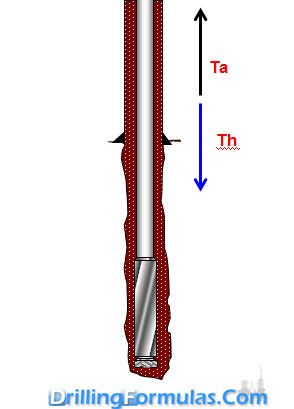# Margin of Overpull in Drillstring

Margin of overpull is additional tension to be applied when pulling the stuck drill string without breaking the tensile limit of the drill string. This is the difference between maximum allowable tensile load of drill string and hook load.

There are several factors when consider about the margin of over pull as listed below;

• Overall drilling conditions
• Hole drag
• Likelihood of getting stuckThe formula for margin of overpull is described below;

## Margin of Overpull = Ta – Th

Where;

Ta is the maximum allowable tensile strength, lb.

Th is the hook load (excluding top drive weight), lb.

Th can be calculated by the following formula;

Th = Weight of String x Buoyancy Factor (BF)

Weight of string = (Ldp×Wdp) + (Ldc×Wdc) +(Lhwdp×Whwdp)+(Lbha × Wbha)Where;

Ldp = length of drill pipe

Wdp = weight of drill pipe

Ldc = length of drill collar

Wdc= weight of drill collar

Lhwdp = length of heavy weight drill pipe

Whwdp= weight of heavy weight drill pipe

Lbha = length of bottom hole assembly

Wbha= weight of bottom hole assembly

Buoyancy Factor (BF) = (65.5 – MW)÷65.5

The ratio between Ta and Th is safety factor (SF).

## SF =Ta ÷ Th

Example: The drill string consists of the following equipment:

5” DP S-135, 4-1/2” IF connection, adjusted weight of 23.5 ppf = 8,000 ft

5” HWDP S-135, 4-1/2” IF connection, adjusted weight of 58 ppf = 900 ft

Mud motor and MWD weight = 20 Klb

Lenght of mud motor and MWD = 90 ft

Mud weight is 9.2 ppg

Tensile strength of 5” DP S-135 (premium class) = 436 Klb

Tensile strength of 5” HWDP S-135 (premium class) = 1,100 Klb

90% of tensile strength is allowed to pull without permission from town.

Determine the margin of overpull from the information above.

Maximum tension will happen at the surface so 5”DP will get the most tension when pulling and since only 90% of tensile strength is allowed. The allowable tensile (Ta) is as follows;

Ta = 0.9 x 436 = 392 Klb

Buoyancy Factor (BF) = (65.5 – 9.2)÷65.5

Buoyancy Factor (BF) = 0.86

Weight of string = (8,000 × 23.5) + (900 × 58) +  (20,000)

Weight of string = 260 Klb

Note: Weight of mud motor and MWD is given at 20Klb.

Tension on surface (Th)

Th = 260 × 0.86 = 223.8 klb

Margin of over pull = 392 – 223.8 = 168.2 Klb

Safety Factor = 392 ÷ 223.8 = 1.75

Share the joy
Tagged , . Bookmark the permalink.Working in the oil field and loving to share knowledge.

### 26 Responses to Margin of Overpull in Drillstring

1.Ahmed Moustafa says:

thanks for teaching us all these informations

2.Hamid says:

Thank you Sir for this simple formula
I think you know what I mean .
Best Regards

3.Ahmad Jafari says:

Thanks for teaching us all these informations

4.khairy says:

thanks for sharing knowledge
I have question what the over-pull limit while round trip?

•DrillingFormulas.Com says:

You have limitation at the weakest point in while you trip out. You need to check pipe tensile strength and determine how much it is.

5.Nasser says:

Thanks that is usefull

6.Ali says:

Excellent side to improve skills.I really appriciated.
in this evaquation (Margin of overpull…….where get 0.9

7.hussein hazawy says:

What is the unit of safety factor here

•DrillingFormulas.Com says:

The unit is %.

•Sun Tzu says:

It’s not %
Ratio does not have units.

8.hussein says:

Margin over pull =(TR we ×TSF)+(((DC L×DCwf)+(HW L ×HW wf ) +(WP wf ×BF×WP L)))

9.Fouad says:

what about the mixed string of 3 1/2″ drill pipe and 5″ G105 and 5″ S135 how we can calculate the maximum marten Decker Reading

•DrillingFormulas.Com says:

You need to do free body diagram in order to understand the load distribution because yield strength of each pipe is not the same value.

Cheers,
Shyne

10.Mohammed says:

Can you please tell me the proper torque have to give to drill string prior jar down?
drill pipe= 5.5″, 4-1/2″ IF, 19.5 ppf

•DrillingFormulas.Com says:

4-1/2″ connection should be made up to 30K. This will be good for jarring operation too.

11.Mohamed Ali says:

Hi,
Sorry for the delay.I niticed that you didn’t concider the mud weight buoncy factor( sorry in this case the hook weight is already given , this is only to remind my friends)

•DrillingFormulas.Com says:

Mud weight has been considered in the calculation.

12.El Atanor says:

In this approach torque effect is neglected. Torque applied reduces string´s max. tensile strenght. When applying both tension and torque you should calculate which is new Ta. Combined forces.

13.Jorge Saltos says:

Excelente publicacion, gracias por su informacion

14.piyush k sushama says:

Thanks, It is very useful / informative for drilling personnel

regards
piyush

15.Sun Tzu says:

In the example problem, “Mud motor and MWD weight = 20 Klb”
but in the calculation “20Klb” is not converted to “lb” : “Weight of string = (8000 × 23.5) + (900 × 58) + (20)”
Please fix to :(8000 × 23.5) + (900 × 58) + (20000)
or
Mud motor and MWD weight = 20 lb

•DrillingFormulas.Com says:

Sun,

Thanks for spotting my typo mistake. It was fixed.

16.Jimmy Black says:

I am lost with your string weight calculation (8000 x 23.5 DP) + (900 x 58 HW) = 240,200 lbs + (20,000 Motor & MWD) = 260,200 lbs in air.
Then the next sentence states that the motor & MWD are 90Klbs

17.Hussain says:

Hi,
I noticed the mud motor and MWD were not added to the weight of string is that right?
Weight of string = (8000 × 23.5) + (900 × 58) + (20000)

Weight of string = 260 klb not 240 klb

•DrillingFormulas.Com says:

Hussain,

Thanks for information and information is updated.

18.Roberto ruiz gonzalez says:

Gracias camaradas por mantenernos al tanto en las operaciones de riesgo continuo

This site uses Akismet to reduce spam. Learn how your comment data is processed.